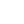# Relaxation

Each point has a known distance so far, if a vertex + distance to a particular point is shorter than the known distance of the point, update it. if (d[v] > d[u] + w(u, v)) d[v] = d[u] + w(u, v)

# Dijkstra Algorithm

Extract min from the priority queue and relax its surroudning vertexes.

The priority queue is implement with Fibonacci heap

```  Initialize-single-source(G, S) // set all distance to infinity
S = {} // path
Q = G.V
while (Q is not empty)
u = EXTRACT-MIN(Q)
S union u
for each vertex v in adj[u] // total E times
RELAX(u, v, w)
```

## Running time

while loop executes V times EXTRACT-MIN take O(log V) decrease key operation O(1) with total edges E times

Overall Running time: `O(V log V + E)`

Naive method: O(V^2 + E), (linear search for EXTRACT-MIN)

## Limitation

Only applicable for graphs with positive edge

# Bellman-Ford Algorithm

Suppose a path from source to destination at most contains V - 1 edges It basically try to relax every edges in the path until you reach the destination It is based on dynamic proramming, but without a successor table of each vertex. d[i, v] = d[i - 1, v] d[i, v] = min(d[i, v], min(i - 1, u) + w(u, v))

```for i = 1 to V - 1
for each vertex u
if (d[u] is updated)
for each vertex v in adj[u]
if (d[v] > d[u] + w(u, v))
d[v] = d[u] + w(u, v)
successor = u
if no value is change, break
```

It can also detect negative cycle

```  for each edge(u, v)
if (d[u] + w(u, v) < d[v])
"negative cycle detected !!"
```

## Running time analysis

Simply O(VE), memory space (V + E)